# 0708-1300/Class notes for Thursday, November 1

Announcements go here

## Today's Agenda

• HW4 and TE1.
• Continue with Tuesday's agenda:
• Debt on proper functions.
• Prove that "the sphere is not contractible".
• Complete the proof of the "tubular neighborhood theorem".

### Proper Implies Closed

Theorem. A proper function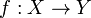$f:X\to Y$ from a topological space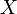$X$ to a locally compact (Hausdorff) topological space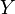$Y$ is closed.

Proof. Let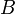$B$ be closed in$X$, we need to show that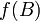$f(B)$ is closed in$Y$. Since closedness is a local property, it is enough to show that every point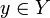$y\in Y$ has a neighbourhood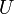$U$ such that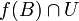$f(B)\cap U$ is closed in$U$. Fix$y\in Y$, and by local compactness, choose a neighbourhood$U$ of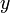$y$ whose close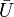$\bar U$ is compact. Then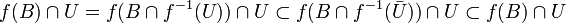$f(B)\cap U=f(B\cap f^{-1}(U))\cap U\subset f(B\cap f^{-1}(\bar U))\cap U\subset f(B)\cap U$,

so that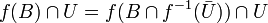$f(B)\cap U=f(B\cap f^{-1}(\bar U))\cap U$. But$\bar U$ is compact by choice, so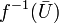$f^{-1}(\bar U)$ is compact as$f$ is proper, so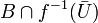$B\cap f^{-1}(\bar U)$ is compact as$B$ is closed, so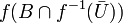$f(B\cap f^{-1}(\bar U))$ is compact (and hence closed) as a continuous image of a compact set, so$f(B)\cap U$ is the intersection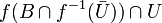$f(B\cap f^{-1}(\bar U))\cap U$ of a closed set with$U$, hence it is closed in$U$.

### Note

The example of a non-contractible "comb" seen today is, in fact, "Cantor's comb". See, for example, page 25 of www.karlin.mff.cuni.cz/~pyrih/e/e2000v0/c/ect.ps

### Typed Notes

Definition 1

X is contractible to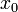$x_0$ if there exists a continuous function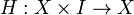$H:X\times I\rightarrow X$ where

1)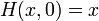$H(x,0) = x$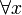$\forall x$

2)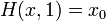$H(x,1) = x_0$$\forall x$

3)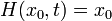$H(x_0,t)=x_0$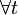$\forall t$

Example 1

The singleton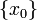$\{x_0\}$ is contractible

Example 2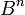$B^n$,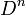$D^n$ and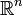$\mathbb{R}^n$ are all contractible. For instance,$\mathbb{R}^n$ is contractible via the function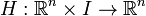$H:\mathbb{R}^n\times I\rightarrow\mathbb{R}^n$ given by H(x,t) = (1-t)x.

Example 3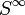$S^{\infty}$ [[0708-1300/the unit sphere in a Hilbert space is contractible

the unit sphere in a Hilbert space is contractible]]


Example 4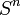$S^n$ is NOT contractible for all n (as we shall prove)

Example 5

Consider the Cantor Comb consisting of the subset of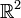$\mathbb{R}^2$ consisting of the unit interval along the x axis with a spike of height 1 going up perpendicularly to the x axis at the location 1/n for n = 0,1,2,...

This set is contractible to (0,0) via the mapping that shrinks all the spikes on the comb to the real axis in time t=1/2 and then shrinks the interval to the point (0,0) by time t=1.

However, this set is NOT contrabible to (0,1). This is because should we try to flatten to the real axis points on the teeth of the comb within an epsilon neighborhood of (1,0) they would pull (1,0) down to the real axis as well otherwise continuity would be broken. Hence the third requirement of a contraction is broken.

Proposition$S^n$ is not contractible

Assume not, thus it is contractible with contraction H(x,t).

Consider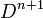$D^{n+1}$ and consider the spherical shell of diameter less than or equal to 1 inside$D^{n+1}$ where each shell comes to rest tangentially to a point$x_0$ on$S^n$. This is like the idea of making a new spherical shell for each such diameter inside of$D^{n+1}$ and letting all the shells "fall" to the bottom.

We now define a retract r on$D^{n+1}$ by associating H(-,t) with the spherical shell of diameter t. I.e. the outside shell of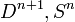$D^{n+1}, S^{n}$ is associated with H(x,0).

Hence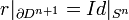$r|_{\partial D^{n+1}} = Id|_{S^n}$. R is clearly continuous and is a retract. But we proved last class that such retracts are impossible and this establishes the contradiction.

Q.E.D